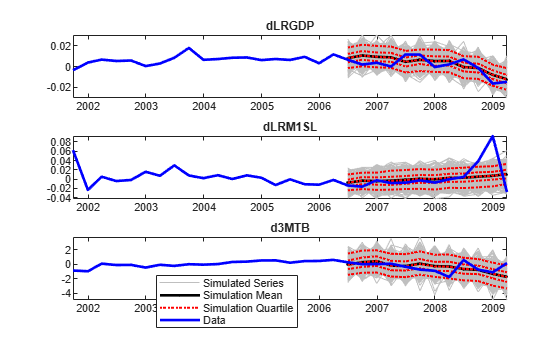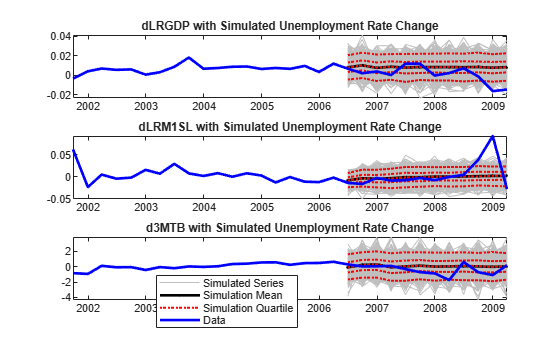Documentation

## Simulate Responses of Estimated VARX Model

This example shows how to estimate a multivariate time series model that contains lagged endogenous and exogenous variables, and how to simulate responses. The response series are the quarterly:

• Changes in real gross domestic product (rGDP) rates (${y}_{1t}$)

• Real money supply (rM1SL) rates (${y}_{2t}$)

• Short-term interest rates (i.e., three-month treasury bill yield, ${y}_{3t}$)

from March, 1959 through March, 2009 . The exogenous series is the quarterly changes in the unemployment rates (${x}_{t}$).

Suppose that a model for the responses is this VARX(4,3) model

`$\left[\begin{array}{c}{y}_{1t}\\ {y}_{2t}\\ {y}_{3t}\end{array}\right]=\left[\begin{array}{c}{a}_{1}\\ {a}_{2}\\ {a}_{3}\end{array}\right]+\left[\begin{array}{ccc}{x}_{t}& 0& 0\\ 0& {x}_{t}& 0\\ 0& 0& {x}_{t}\end{array}\right]\left[\begin{array}{c}{b}_{1}\\ {b}_{2}\\ {b}_{3}\end{array}\right]+\left[\begin{array}{ccc}{\varphi }_{11}^{\left(1\right)}& {\varphi }_{12}^{\left(1\right)}& {\varphi }_{13}^{\left(1\right)}\\ {\varphi }_{21}^{\left(1\right)}& {\varphi }_{22}^{\left(1\right)}& {\varphi }_{23}^{\left(1\right)}\\ {\varphi }_{31}^{\left(1\right)}& {\varphi }_{32}^{\left(1\right)}& {\varphi }_{33}^{\left(1\right)}\end{array}\right]\left[\begin{array}{c}{y}_{1,t-1}\\ {y}_{2,t-1}\\ {y}_{3,t-1}\end{array}\right]+...+\left[\begin{array}{ccc}{\varphi }_{11}^{\left(4\right)}& {\varphi }_{12}^{\left(4\right)}& {\varphi }_{13}^{\left(4\right)}\\ {\varphi }_{21}^{\left(4\right)}& {\varphi }_{22}^{\left(4\right)}& {\varphi }_{23}^{\left(4\right)}\\ {\varphi }_{31}^{\left(4\right)}& {\varphi }_{32}^{\left(4\right)}& {\varphi }_{33}^{\left(4\right)}\end{array}\right]\left[\begin{array}{c}{y}_{1,t-1}\\ {y}_{2,t-1}\\ {y}_{3,t-1}\end{array}\right]+\left[\begin{array}{c}{\epsilon }_{1t}\\ {\epsilon }_{2t}\\ {\epsilon }_{3t}\end{array}\right]$`

### Preprocess the Data

Load the U.S. macroeconomic data set. Flag the series and their periods that contain missing values (indicated by `NaN` values).

```load Data_USEconModel varNaN = any(ismissing(DataTable),1); % Variables containing NaN values seriesWithNaNs = series(varNaN)```
```seriesWithNaNs = 1x5 cell array Columns 1 through 3 {'(FEDFUNDS) Eff...'} {'(GS10) Ten-yea...'} {'(M1SL) M1 mone...'} Columns 4 through 5 {'(M2SL) M2 mone...'} {'(UNRATE) Unemp...'} ```

In this data set, the variables that contain missing values entered the sample later than the other variables. There are no missing values after sampling started for a particular variable.

Flag all periods corresponding to missing values in the model variables.

`idx = all(~ismissing(DataTable(:,{'UNRATE' 'M1SL'})),2);`

For the rest of the example, consider only those values that of the series indicated by a `true` in `idx`.

Compute rGDP and rM1SL, and the growth rates of rGDP, rM1SL, short-term interest rates, and the unemployment rate. `Description` contains a description of the data and the variable names. Reserve the last three years of data to investigate the out-of-sample performance of the estimated model.

```rGDP = DataTable.GDP(idx)./(DataTable.GDPDEF(idx)/100); rM1SL = DataTable.M1SL(idx)./(DataTable.GDPDEF(idx)/100); dLRGDP = diff(log(rGDP)); % rGDP growth rate dLRM1SL = diff(log(rM1SL)); % rM1SL growth rate d3MTB = diff(DataTable.TB3MS(idx)); % Change in short-term interest rate (3MTB) dUNRATE = diff(DataTable.UNRATE(idx)); % Change in unemployment rate T = numel(d3MTB); % Total sample size oosT = 12; % Out-of-sample size estT = T - oosT; % Estimation sample size estIdx = 1:estT; % Estimation sample indices oosIdx = (T - 11):T; % Out-of-sample indices dates = dates((end - T + 1):end); EstY = [dLRGDP(estIdx) dLRM1SL(estIdx) d3MTB(estIdx)]; % In-sample responses estX = dUNRATE(estIdx); % In-sample exogenous data n = size(EstY,2); OOSY = [dLRGDP(oosIdx) dLRM1SL(oosIdx) d3MTB(oosIdx)]; % Out-of-sample responses oosX = dUNRATE(oosIdx); % Out-of-sample exogenous data```

### Create VARX Model

Create a VARX(4) model using `varm`.

`Mdl = varm(n,4);`

`Mdl` is a `varm` model object serving as a template for estimation. Currently, `Mdl` does know have the structure in place for the regression component. However, MATLAB® creates the required structure during estimation.

### Estimate the VAR(4) Model

Estimate the parameters of the VARX(4) model using `estimate`. Display the parameter estimates.

```EstMdl = estimate(Mdl,EstY,'X',estX); summarize(EstMdl)```
``` AR-Stationary 3-Dimensional VARX(4) Model with 1 Predictor Effective Sample Size: 184 Number of Estimated Parameters: 42 LogLikelihood: 1037.52 AIC: -1991.04 BIC: -1856.01 Value StandardError TStatistic PValue ___________ _____________ __________ __________ Constant(1) 0.0080266 0.00097087 8.2674 1.3688e-16 Constant(2) 0.00063838 0.0015942 0.40044 0.68883 Constant(3) 0.068361 0.143 0.47803 0.63263 AR{1}(1,1) -0.034045 0.06633 -0.51327 0.60776 AR{1}(2,1) -0.0024555 0.10891 -0.022546 0.98201 AR{1}(3,1) -1.7163 9.77 -0.17567 0.86056 AR{1}(1,2) -0.013882 0.046481 -0.29867 0.76519 AR{1}(2,2) 0.17753 0.076323 2.326 0.020017 AR{1}(3,2) -6.7572 6.8464 -0.98697 0.32366 AR{1}(1,3) 0.0010682 0.00048092 2.2212 0.026337 AR{1}(2,3) -0.0050252 0.00078967 -6.3636 1.9705e-10 AR{1}(3,3) -0.16256 0.070837 -2.2948 0.021744 AR{2}(1,1) 0.077748 0.064014 1.2145 0.22454 AR{2}(2,1) 0.0047257 0.10511 0.044959 0.96414 AR{2}(3,1) 3.4244 9.4289 0.36318 0.71647 AR{2}(1,2) 0.077867 0.046954 1.6584 0.097245 AR{2}(2,2) 0.29087 0.077099 3.7727 0.00016148 AR{2}(3,2) 0.39284 6.9161 0.0568 0.9547 AR{2}(1,3) -0.0010719 0.00056413 -1.9001 0.057423 AR{2}(2,3) -0.0016135 0.00092631 -1.7419 0.081533 AR{2}(3,3) -0.21556 0.083094 -2.5942 0.0094802 AR{3}(1,1) -0.090881 0.062563 -1.4526 0.14633 AR{3}(2,1) 0.064249 0.10273 0.62542 0.53169 AR{3}(3,1) -7.9727 9.2152 -0.86517 0.38695 AR{3}(1,2) -0.024092 0.04631 -0.52024 0.60289 AR{3}(2,2) 0.068565 0.076041 0.90168 0.36723 AR{3}(3,2) 10.263 6.8212 1.5046 0.13242 AR{3}(1,3) -0.00055981 0.00056073 -0.99836 0.31811 AR{3}(2,3) -0.0021302 0.00092073 -2.3136 0.02069 AR{3}(3,3) 0.22969 0.082593 2.7809 0.0054203 AR{4}(1,1) 0.066151 0.056841 1.1638 0.24451 AR{4}(2,1) 0.028826 0.093334 0.30885 0.75744 AR{4}(3,1) 1.0379 8.3724 0.12397 0.90134 AR{4}(1,2) -0.078735 0.043804 -1.7975 0.072263 AR{4}(2,2) 0.0096425 0.071926 0.13406 0.89335 AR{4}(3,2) -12.007 6.4521 -1.8609 0.062761 AR{4}(1,3) -0.00018454 0.00053356 -0.34586 0.72945 AR{4}(2,3) -0.00019036 0.00087611 -0.21728 0.82799 AR{4}(3,3) 0.053812 0.078591 0.68471 0.49353 Beta(1,1) -0.016084 0.0016037 -10.029 1.1365e-23 Beta(2,1) -0.00154 0.0026333 -0.58482 0.55867 Beta(3,1) -1.5317 0.23622 -6.4841 8.9252e-11 Innovations Covariance Matrix: 0.0000 0.0000 0.0000 0.0000 0.0001 -0.0019 0.0000 -0.0019 0.7790 Innovations Correlation Matrix: 1.0000 0.1198 0.0011 0.1198 1.0000 -0.2177 0.0011 -0.2177 1.0000 ```

`EstMdl` is a `varm` model object containing the estimated parameters.

### Simulate Out-Of-Sample Response Paths

Simulate 1000, 3 year response series paths from the estimated model assuming that the exogenous unemployment rate is a fixed series. Since the model contains 4 lags per endogenous variable, specify the last 4 observations in the estimation sample as presample data.

```numPaths = 1000; Y0 = EstY((end-3):end,:); rng(1); % For reproducibility YSim = simulate(EstMdl,oosT,'X',oosX,'Y0',Y0,'NumPaths',numPaths);```

`YSim` is a 12-by-3-by-1000 numeric array of simulated responses. The rows of `YSim` correspond to out-of-sample periods, the columns correspond to the response series, and the pages correspond to paths.

Plot the response data and the simulated responses. Identify the 5%, 25%, 75% and 95% percentiles, and the mean and median of the simulated series at each out-of-sample period.

```YSimBar = mean(YSim,3); YSimQrtl = quantile(YSim,[0.05 0.25 0.5 0.75 0.95],3); RepDates = repmat(dates(oosIdx),1,1000); respNames = {'dLRGDP' 'dLRM1SL' 'd3MTB'}; figure; for j = 1:n subplot(3,1,j); h1 = plot(dates(oosIdx),squeeze(YSim(:,j,:)),'Color',0.75*ones(3,1)); hold on; h2 = plot(dates(oosIdx),YSimBar(:,j),'.-k','LineWidth',2); h3 = plot(dates(oosIdx),squeeze(YSimQrtl(:,j,:)),':r','LineWidth',1.5); h4 = plot(dates((end - 30):end),[EstY((end - 18):end,j);OOSY(:,j)],... 'b','LineWidth',2); title(sprintf('%s',respNames{j})); datetick; axis tight; hold off; end legend([h1(1) h2(1) h3(1) h4],{'Simulated Series','Simulation Mean',... 'Simulation Quartile','Data'},'Location',[0.4 0.1 0.01 0.01],... 'FontSize',8);```### Simulate Out-Of-Sample Response Paths Using Random Exogenous Data

Suppose that the change in the unemployment rate is an AR(4) model, and fit the model to the estimation sample data.

```MdlUNRATE = arima('ARLags',1:4); EstMdlUNRATE = estimate(MdlUNRATE,estX,'Display','off');```

`EstMdlUNRATE` is an `arima` model object containing the parameter estimates.

Simulate 1000, 3 year paths from the estimated AR(4) model for the change in unemployment rate. Since the model contains 4 lags, specify the last 4 observations in the estimation sample as presample data.

```XSim = simulate(EstMdlUNRATE,oosT,'Y0',estX(end-3:end),... 'NumPaths',numPaths);```

`XSim` is a 12-by-1000 numeric matrix of simulated exogenous paths. The rows correspond to periods and the columns correspond to paths.

Simulate 3 years of 1000 future response series paths from the estimated model using the simulated exogenous data. `simulate` does not accept multiple paths of predictor data, so you must simulate responses in a loop. Since the model contains 4 lags per endogenous variable, specify the last 4 observations in the estimation sample as presample data.

```YSimRX = zeros(oosT,n,numPaths); % Preallocate for j = 1:numPaths YSimRX(:,:,j) = simulate(EstMdl,oosT,'X',XSim(:,j),'Y0',Y0); end```

`YSimRX` is a 12-by-3-by-1000 numeric array of simulated responses.

Plot the response data and the simulated responses. Identify the 5%, 25%, 75% and 95% percentiles, and the mean and median of the simulated series at each out-of-sample period.

```YSimBarRX = mean(YSimRX,3); YSimQrtlRX = quantile(YSimRX,[0.05 0.25 0.5 0.75 0.95],3); figure; for j = 1:n; subplot(3,1,j); h1 = plot(dates(oosIdx),squeeze(YSimRX(:,j,:)),'Color',0.75*ones(3,1)); hold on; h2 = plot(dates(oosIdx),YSimBarRX(:,j),'.-k','LineWidth',2); h3 = plot(dates(oosIdx),squeeze(YSimQrtlRX(:,j,:)),':r','LineWidth',1.5); h4 = plot(dates((end - 30):end),[EstY((end - 18):end,j);OOSY(:,j)],... 'b','LineWidth',2); title(sprintf('%s with Simulated Unemployment Rate',respNames{j})); datetick; axis tight; hold off; end legend([h1(1) h2(1) h3(1) h4],{'Simulated Series','Simulation Mean',... 'Simulation Quartile','Data'},'Location',[0.4 0.1 0.01 0.01],... 'FontSize',8)```